Courses

# Thermodynamic Potential MSQ

## 10 Questions MCQ Test Topic wise Tests for IIT JAM Physics | Thermodynamic Potential MSQ

Description
This mock test of Thermodynamic Potential MSQ for Physics helps you for every Physics entrance exam. This contains 10 Multiple Choice Questions for Physics Thermodynamic Potential MSQ (mcq) to study with solutions a complete question bank. The solved questions answers in this Thermodynamic Potential MSQ quiz give you a good mix of easy questions and tough questions. Physics students definitely take this Thermodynamic Potential MSQ exercise for a better result in the exam. You can find other Thermodynamic Potential MSQ extra questions, long questions & short questions for Physics on EduRev as well by searching above.
*Multiple options can be correct
QUESTION: 1

### A fluid at high pressure is throttled through a narrow porous opening in a region of lower pressure without any transfer of heat. In such a process which of the following is not correct. Select one or more:

Solution:

Since there is no transfer of heat, the enthalpy of the fluid remain constant, everything else changes in the process.
The correct answers are: The entropy does not change, The Gibb’s free energy remains constant, The entropy is decreased

*Multiple options can be correct
QUESTION: 2

### For liquifying gas, it is essential that the gas Select one or more:

Solution:

Processes like Linde’s  process for air liquefaction need a positive Joule Thomson coefficient, hence we can only operate at temperature below the inversion temperature. Similarly, we know that above the critical temperature, gas cannot be liquified by varying the pressure, therefore the temperature of the gas should be below critical temperature.
The correct answers are: Should be below its temperature of inversion, Should be below the critical temperature

*Multiple options can be correct
QUESTION: 3

### Gibb’s thermodynamical potential can be represented as G = H – TS  (symbols have their usual meaning), which relation holds true? Select one or more:

Solution:

G = H – TS
dG = dH – TdS – SdT
dG = dU + pdV + Vdp – TdS – SdT
Further dG = –SdT + Vdp + µdN
⇒ dU – TdS = µdN + pdV
⇒ TdS = dV – µdN + pdV
Keeping V and N constant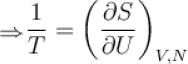Keeping U, V constant.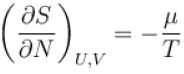The correct answers are: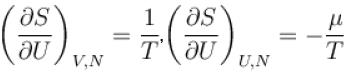*Multiple options can be correct
QUESTION: 4

Which among the following for Maxwell’s relation are correct?
(U - internal energy, H - enthalpy, S - entropy)
Select one or more:

Solution:

We know that     H = U + pV
⇒ dH = dV + pdV + Vdp
and dV = TdS + VdP
⇒ dH = TdS + Vdp
Now keeping p as constant,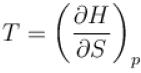keeping S as constant,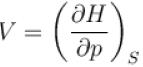The correct answers are: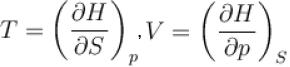*Multiple options can be correct
QUESTION: 5

According to consequences of the second law of thermodynamics (symbols have their usual meaning)
Select one or more:

Solution:

From second law of thermodynamics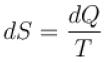and dQ = dU + pdV

⇒ TdS = dU + pdV
⇒ dU = TdS – pdV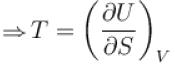and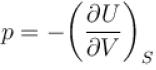The correct answers are: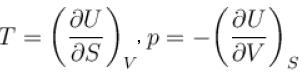*Multiple options can be correct
QUESTION: 6

Which of the following statements are true?
Select one or more:

Solution:

A massive ideal gas with only one type of particle is uniquely described by three state functions such as temperature, volume and number of particles. However for a black body, the energy distribution is established by interaction of photons with matter, usually walls of container. In this interaction, number of photons is not conserved. As a result, chemical potential is zero.
Further (b) and (c) are basic facts about the chemical potential and hence are true.
The correct answers are: Chemical potential for photon is zero, In phase equilibrium, total sum of chemical potentials is zero, Chemical potential of electron at zero Kelvin is same as Fermi Energy.

*Multiple options can be correct
QUESTION: 7

Denoting temperature, entropy and internal energy by T, S and U respectively and their initial and final values by subscript i and f respectively, the Joule Thomson expansion of an ideal gas can be expressed as
Select one or more:

Solution:

In Joule-Thomson expansion of an ideal gas, internal energy and temperature remains same but entropy increases hence (a), (c) and (d) are correct options.
The correct answers are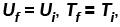*Multiple options can be correct
QUESTION: 8

Which of the following are correct terms of Maxwell’s relation
Select one or more:

Solution:

Except option (c), all the other options give one of Maxwell’s equation and the fourth missing equal is given as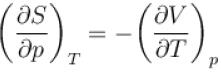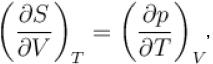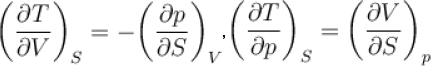*Multiple options can be correct
QUESTION: 9

For an isolated thermodynamical system p, V, T, U, S and F represent the pressure, volume, temperature, internal energy,entropy and free energy respectively. Then, following relation are true
Select one or more:

Solution:

Since      F = U – TS
dF = TdS – pdU – TdS – SdT
keeping V constant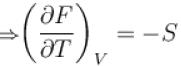Also, dU = TdS – pdV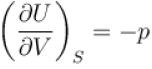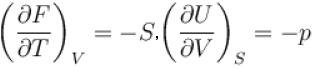*Multiple options can be correct
QUESTION: 10

For a thermodynamic system Helmholtz free energy is a function of
Select one or more:

Solution:

F = U – TS
⇒ dF = dU – TdS – SdT
dF = TdS – pdV – TdS – SdT
⇒ dF = –pdV – SdT
⇒ F is a function of V and T.